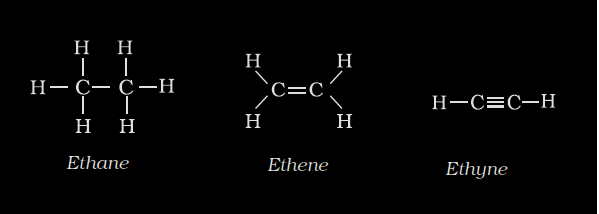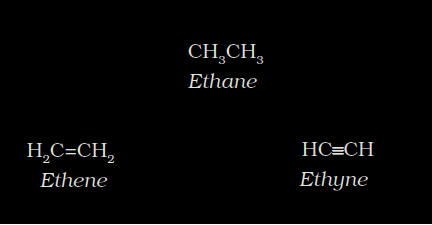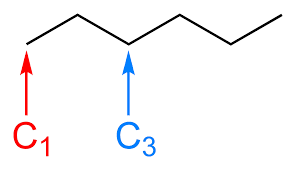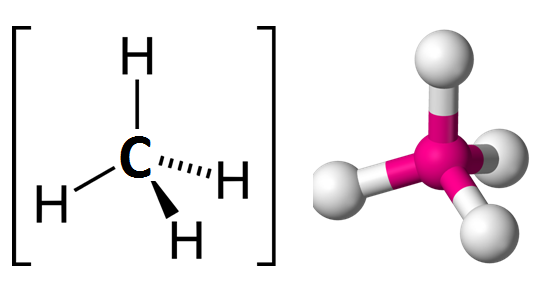# Organic Compounds: Structural Representation

### What is an Organic Compound?

Carbon is known for its catenation property. The covalently bonded carbon-containing compounds called organic compounds are substantial for life on earth. The specific branch of science that deals with organic compounds are called organic chemistry. Organic chemistry encompasses every characteristic feature of an organic compound, its properties, and behaviour under different conditions. Here we will discuss the structural representation of organic molecules.

#### Structural Representation of Organic Compounds:

Though an organic compound has only one chemical formula, structurally it can be depicted in numerous ways. The three structural formulas – complete structure, condensed structure, and bond line structural formulas are explained below.

#### Complete Structural Formula:

The Lewis dot structure is considered as the complete structural formula. In Lewis structure, the covalent bonds in the compound are denoted by a dash (―). This helps to emphasize the number of bonds formed by the electrons. Every single bond, a double bond, and a triple bond are represented by one dash, double dash, and triple dash respectively. It illustrates every single bond formed between every atom in the compound, thus called complete structural formula.#### Condensed Structural Formula:

Since complete structural formula consumes much time and space to represent the structure, we can condense them. This is the condensed structural formula, where replacing some dashes/bonds by a number of identical groups attached to an atom by a subscript.#### Bond Line Structural Formula:

A bond lines structural formula is another way of structural representation of organic compounds.  Here, every bond is represented as a line in a zigzag manner. If not specified, every terminal is assumed to be a methyl (-CH3) group.Bond Line Structural Formula#### 3-D Representation of Organic Compounds:

The organic compounds which were represented using structural formulas in the previous section are three-dimensional compounds. In order to draw the 3-D structure of an organic compound, we can use wedge-dash representation.

The bond that protrudes out of the plane of paper towards the viewer is denoted by a solid wedge while that project away from the viewer or into the plane of the paper is denoted by a dashed wedge and the bond in the plane of the paper is represented by a line.3-D Representation of Organic Compounds – Methane